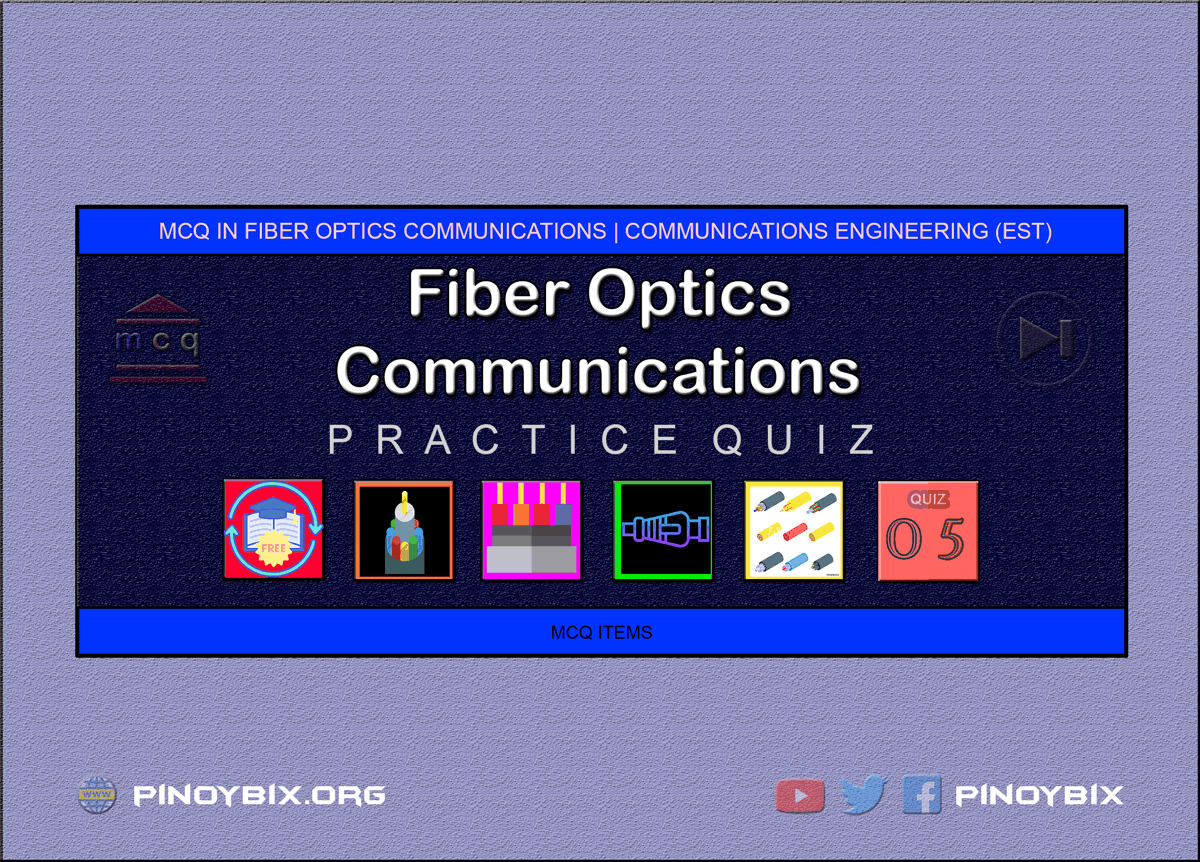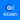# MCQ in Fiber Optics Communications Part 5 | ECE Board Exam

(Last Updated On: April 27, 2020)This is the Multiples Choice Questions Part 5 of the Series in Fiber Optics Communications as one of the Communications Engineering topic. In Preparation for the ECE Board Exam make sure to expose yourself and familiarize in each and every questions compiled here taken from various sources including but not limited to past Board Examination Questions in Electronic System and Technologies, Communications Books, Journals and other Communications References.

#### MCQ Topic Outline included in ECE Board Exam Syllabi

• MCQ in Principles of Light Transmission
• MCQ in Types of Light Sources, Laser, LED
• MCQ in Light Detectors
• MCQ in Modulation and Waveform
• MCQ in System Design
• MCQ in General application
• MCQ in System Bandwidth
• MCQ in Splicing Techniques

#### Continue Practice Exam Test Questions Part 5 of the Series

201. In refraction that occurs in air/glass interfaces, among the visible light, which is the bent the least?

A. violet

B. blue

C. red

D. orange

Solution:

202. Ratio of the velocity of propagation of a light ray in free space to the velocity of propagation of a light ray in free space in a given material.

A. refractive index

B. standing wave ratio

C. velocity factor

D. propagation velocity

Solution:

203. It is the angle at which the propagating ray strikes the interface with respect to the normal.

A. refracted angle

B. incident angle

C. reflected angle

D. critical angle

Solution:

204. It is the angle formed between the propagating ray and the normal after the ray has entered the second medium.

A. angle of incidence

B. angle of reflection

C. propagation angle

D. angle of refraction

Solution:

205. Between silicon and gallium arsenide, which has the greatest index of refraction?

A. Gallium arsenide

B. Neither of silicon nor gallium arsenide

C. Silicon

D. They are equal

Solution:

206. A fiber-optic cable has a loss of 15 dB/km. The attenuation in a cable 1000 ft. long is

A. 4.57 dB

B. 9.3 dB

C. 24 dB

D. 49.2 dB

Solution:

207. Medium 1 is a glass (n1 = 1.5) and medium 2 is an ethyl alcohol (n2 = 1.36). For an angle of incidence of 30 degrees, determine the angle of refraction.

A. 44.5 degrees

B. 14.56 degrees

C. 33.47 degrees

D. 75 degrees

Solution:

208. The minimum angle of incidence at which the light ray may strike the interface of two media and result in an angle of refraction of 90 degrees or greater.

A. optimum angle

B. angle of refraction

C. refracted angle

D. critical angle

Solution:

209. The higher the index number

A. the higher the speed of light

B. the lower the speed of light

C. has no effect on the speed of light

D. the shorter the wavelength propagation

Solution:

210. The maximum angle in which external light rays may strike the air/glass interface and still propagate down the fiber.

A. Acceptance cone half-angle

B. Acceptance cone

C. Critical angle

D. Angle of incidence

Solution:

211. It is the figure of merit used to measure the magnitude of the acceptance angle.

A. acceptance angle

B. numerical aperture

C. index profile

D. refractive index

Solution:

212. The effect of a large magnitude of the numerical aperture

A. The amount of external light the fiber will accept is greater.

B. The amount of external light the fiber will accept is less.

C. The amount of modal dispersion will be less.

D. The amount of chromatic dispersion will be greater.

Solution:

213. Only one path for light rays to take down the fiber

A. Multimode

B. Step-index

C. Single mode

Solution:

214. More than one path for light rays to take down the fiber

A. Multimode

B. Step-index

C. Single mode

Solution:

215. The three major groups of the optical system are

A. the components, the data rate and the response time

C. the transmitter, the cable and the receiver

D. the source, the link and the detector

Solution:

216. Infrared light has a wavelength that is

A. less than 400 nm

B. more than 700 nm

C. less than 700 nm

D. a little over 400 nm

Solution:

217. How many modes possible with a multimode step-index with a core diameter of 50 um, a core refractive index of 1.6, a cladding refractive index of 1.584, and a wavelength of 1300 nm.

A. 456

B. 213

C. 145

D. 372

Solution:

218. It is a graphical representation of the magnitude of the refractive index across the fiber.

A. mode

B. index profile

C. numerical aperture

D. refractive index

Solution:

219. A type of index profile of an optical fiber that has a central core and outside cladding with a uniform refractive index

A. multimode

C. step-index

D. single mode

Solution:

220. A type of index of an optical fiber that has no cladding and whose central core has a non-uniform refractive index.

B. multimode

C. single mode

D. step-index

Solution:

221. Results in reduction in the power of light wave as it travels down the cable.

A. power loss

B. absorption loss

C. resistive loss

D. heat loss

Solution:

222. Which of the following is not a factor in cable light loss?

A. reflection

B. absorption

C. scattering

D. dispersion

Solution:

223. It is analogous to power dissipation to copper cables, impurities in the fiber absorb the light and covert it to heat.

A. power loss

B. absorption loss

C. resistive loss

D. heat loss

Solution:

224. It is caused by valence electrons in the silica material from which the fiber are manufactured.

A. ion resonance absorption

B. infrared absorption

C. ultraviolet absorption

D. visible light absorption

Solution:

225. It is a result of photons of light that are absorbed by the atoms of the glass core molecule.

A. ion resonance absorption

B. infrared absorption

C. ultraviolet absorption

D. visible light absorption

Solution:

226. It is caused by hydroxide ions in the material

A. visible light absorption

B. infrared absorption

C. ultraviolet absorption

D. ion resonance absorption

Solution:

227. Which type of fiber-optic cable has the least modal dispersion?

A. single-mode step-index

B. multimode step-index

Solution:

228. For a single mode optical cable with 0.25 dB/km loss, determine the optical power 100 km from a 0.1-mW light source.

A. -45 dBm

B. -15 dBm

C. -35 dBm

D. –25 dBm

Solution:

229. Light rays that are emitted simultaneously from an LED and propagated down an optical fiber do not arrive at the far end of the fiber at the same time results to

A. intramodal dispersion

B. pulse length dispersion

C. modal dispersion

D. wavelength dispersion

Solution:

230. Chromatic dispersion can be eliminated by __________.

A. using a monochromatic light source

B. using a very small numerical aperture fiber

D. using a very sensitive photo detector

Solution:

231. Type of bend that occurs as a result of differences in thermal contraction rates between the core and the cladding material.

A. Macrobending

B. Microbending

Solution:

232. These bends are caused by excessive pressure and tension and generally occur while fiber are bent during handling or installation.

A. microbending

B. macrobending

D. kinks

Solution:

233. As light is coupled in a multiport deflective device, the power is reduced by

A. 1.5 dB

B. 0.1 dB

C. 0.5 dB

D. 0.001 dB

Solution:

234. It is caused by the difference in the propagation time of light rays that take different paths down the fiber.

A. modal dispersion

B. microbending

C. Rayleigh scattering

D. chromatic dispersion

Solution:

235. How can modal dispersion reduced entirely?

A. Use a graded index fiber

B. Use a single-mode fiber

C. Use a monochromatic light source

D. Use a very sensitive light detector

Solution:

236. It indicates what signal frequencies can be propagated through a given distance of fiber cable.

A. Bandwidth Distance Product

B. Pulse width dispersion

C. Rise time

D. Cutoff frequency

Solution:

237. For a 300-m optical fiber cable with a bandwidth distance product of 600 MHz-km, determine the bandwidth.

A. 5 GHz

B. 1 GHz

C. 2 GHz

D. 3 GHz

Solution:

238. For an optical fiber 10 km long with a pulse spreading constant of 5 ns/km, determine the maximum digital transmission rates using Return to Zero (RZ) and Non-return to Zero (NRZ).

A. 5 Mbps and 10 Mbps

B. 10 Mbps and 5 Mbps

C. 10 Mbps and 20 Mbps

D. 20 Mbps and 10 Mbps

Solution:

239. What is the spectral width of a standard LED?

A. 20 to 40 nm

B. 30 to 50 nm

C. 10 to 30 nm

D. 40 to 60 nm

Solution:

240. What is the spectral width of an ILD?

A. 0.1 nm to 1 nm

B. 2 nm to 5 nm

C. 1 nm to 3 nm

D. 3 nm to 4 nm

Solution:

241. When connector losses, splice losses and coupler losses are added, what is the limiting factor?

A. source power

B. fiber attenuation

C. connector and splice loss

D. detector sensitivity

Solution:

242. A pn-junction diode emits light by spontaneous emission

A. LED

B. APD

C. PIN

D. Zener diode

Solution:

243. Which type of fiber optic cable is best for very high speed data?

A. single-mode step-index

B. multimode step-index

Solution:

244. A measure of conversion efficiency of a photodetector.

A. Efficiency

B. Responsivity

C. Dark current

D. Spectral response

Solution:

245. The leakage current that flows through a photodiode with no light input

A. dark voltage

B. dark impedance

C. dark power

D. dark current

Solution:

246. The time it takes a light induced carrier travel across the depletion region of the semiconductor.

A. dispersion

B. response time

D. transit time

Solution:

247. The range of wavelength values that a given photodiode will respond.

A. spectral response

B. permeance

C. dark current

D. reluctance

Solution:

248. The term responsivity as it applies to a light detector is best described as

A. the time required for the signal to go from 10 to 90 percent of maximum amplitude

B. the ratio of the diode output current to the input optical power

C. the ratio of the input power to output power

D. the ratio of output current to input current

Solution:

249. The minimum optical power a light detector can receive and still produce a usable electrical output signal.

A. light responsivity

B. light sensitivity

C. light collectivity

D. illumination

Solution:

250. Type of lasers that uses a mixture of helium and neon enclosed in glass tube.

A. gas lasers

B. solid lasers

C. semiconductor lasers

D. liquid lasers

Solution:

#### Questions and Answers in Fiber Optics Communications Series

Following is the list of multiple choice questions in this brand new series:

MCQ in Optical Fiber Communications
PART 1: MCQ from Number 1 – 50                        Answer key: PART 1
PART 2: MCQ from Number 51 – 100                   Answer key: PART 2
PART 3: MCQ from Number 101 – 150                 Answer key: PART 3
PART 4: MCQ from Number 151 – 200                 Answer key: PART 4
PART 5: MCQ from Number 201 – 250                 Answer key: PART 5
PART 6: MCQ from Number 251 – 300                 Answer key: PART 6

### Complete List of MCQ in Communications Engineering per topic

Help Me Makes a Difference!

 P inoyBIX educates thousands of reviewers/students a day in preparation for their board examinations. Also provides professionals with materials for their lectures and practice exams. Help me go forward with the same spirit. “Will you make a small gift today via GCASH?”+63 966 459 6474 Option 1 : \$1 USD Option 2 : \$3 USD Option 3 : \$5 USD Option 4 : \$10 USD Option 5 : \$25 USD Option 6 : \$50 USD Option 7 : \$100 USD Option 8 : Other Amount© 2014 PinoyBIX Engineering. © 2019 All Rights Reserved | How to Donate? |